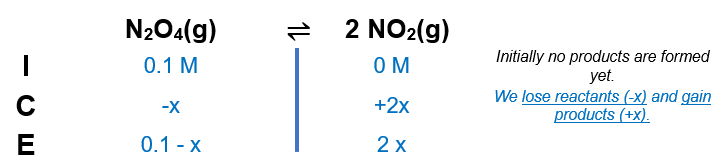# Problem: At a particular temperature, K = 4.0 x 10 -7 for the reactionN2O4(g) ⇌ 2NO2(g)In an experiment, 1.0 mole of N2O4 is placed in a 10.0-L vessel. Calculate the concentrations of N2O4 and NO2 when this reaction reaches equilibrium.

###### FREE Expert Solution

We are asked to calculate the concentrations of N2O4 and NO2 when this reaction reaches equilibrium.

Step 1. Calculate molarity N2O4

M  N2O4 = 0.1 M

Step 2. Set up ICE chartStep 3. Calculate molarity in equilibrium

x is negligible in the denominator since K is very small.

97% (155 ratings)###### Problem Details

At a particular temperature, K = 4.0 x 10 -7 for the reaction

N2O4(g) ⇌ 2NO2(g)

In an experiment, 1.0 mole of N2O4 is placed in a 10.0-L vessel. Calculate the concentrations of N2O4 and NO2 when this reaction reaches equilibrium.